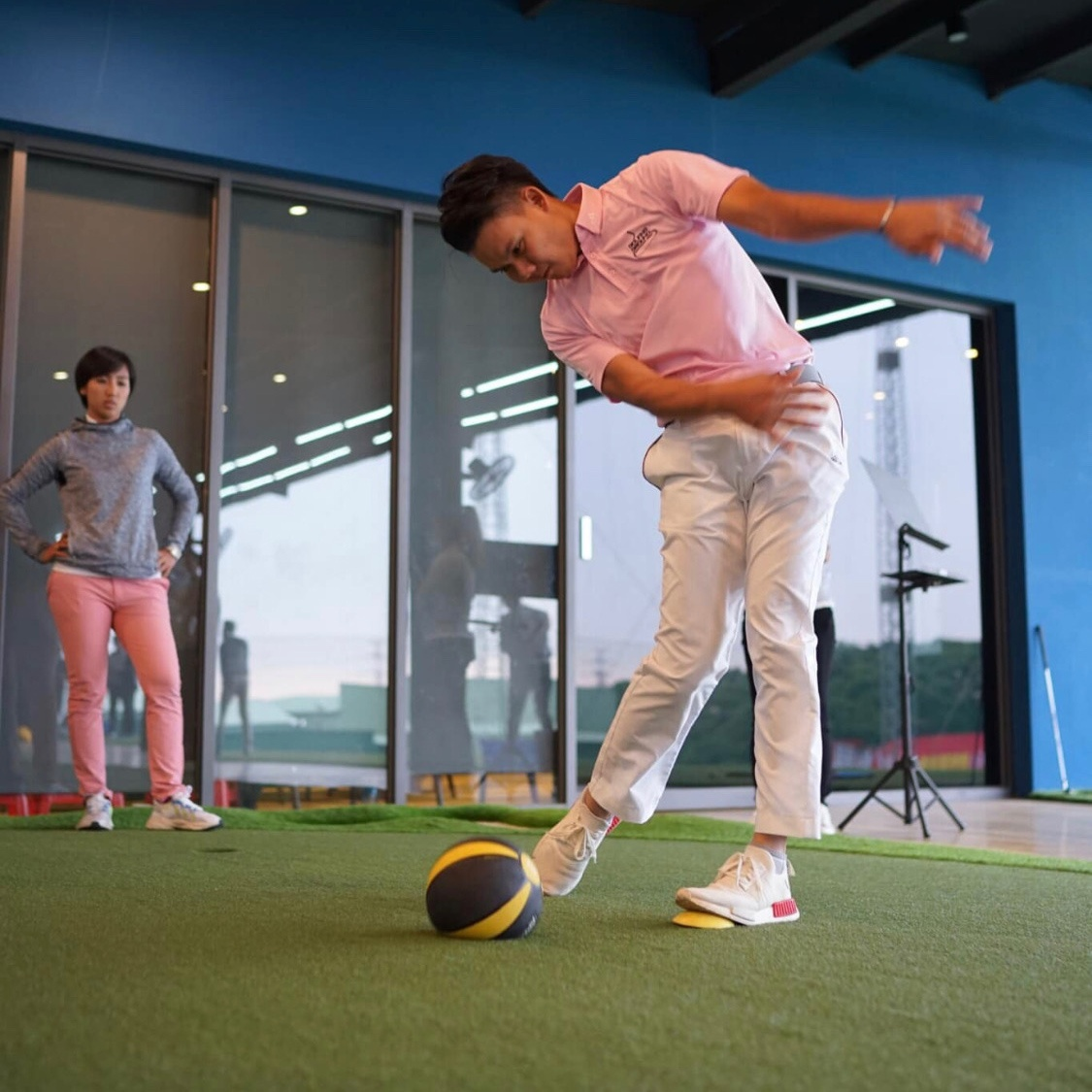Select Page

# Ground reaction force

## Watch and listen Mike talking about ground reaction force and how The Force Pedal and other tools are important to improve your golf swing.« Without those tools, I would be nowhere near the teacher I am. »

## Glossary

### Ground reaction force

In physics, and in particular in biomechanics, the ground reaction force (GRF) is the force exerted by the ground on a body in contact with it. For example, a person standing motionless on the ground exerts a contact force on it and at the same time an equal and opposite ground reaction force is exerted by the ground on the person.

### Force

In physics, a force is an interaction that, when unopposed, will change the motion of an object. In other word, a force can cause an object with mass to change its velocity, that means accelerate (+ or -). Force can also be described by intuitive concept such as a push or a pull. A force has both magnitude and direction, making it a vector quantity. It’s symbol is F, and its unit is newton (N). A force is a power that causes an object to move or that changes movement.

### 1st Newton Law

When viewed in an inertial reference frame, an object either remains at rest or continues to move at a constant velocity, unless acted upon by a force.

### 2nd Newton Law

The vector sum of the forces F on an object is equal to the mass m of that object multiplied by the acceleration vector a of the object:
F = ma.

### 3rd Newton Law

When one body exerts a force on a second body, the second body simultaneously exerts a force equal in magnitude and opposite in direction on the first body.

### Biomechanics

Biomechanics is the study of the structure and function of biological system such as humans, animals, plant, organs and cells by means of the method of mechanics. The study of the effect on physical forces on alive structure and their movement.

### Physiology

Physiology is the scientific study of the normal function in living systems. A sub-discipline of biology, its focus is in how organisms, organ systems, organs, cells and biomolecules carry out the chemical or physical functions that exist in a living system. The scientific study of the way in which the bodies of living things work.

### Center of pressure

In biomechanics, the CoP is the term given to the point of application of the ground reaction force vector. It represent the sum of all forces acting between a physical object and its suporting surface.

### Center of mass

In physics, the center of mass of a distribution of mass in space is the point where the weighted relative position of the distributed mass sums to zero or the point where if a force is applied causes it to move in direction of force without rotation.

### Lateral weight distribution

A way to indicate how your weight is distributed in percentage under left foot and right foot. This distribution on forces indicates in which direction your center of pressure is moving during a golf swing.

### Motion

In physics, motion is a change in position of an object with respect to time. Motion is typically described in terms of displacement, distance, velocity, acceleration, time and speed. Motion of a body is observed by attaching a frame of reference to an observer and measuring the change in position of the body relative to that frame. The act or process of moving. Displacement, distance, velocity, speed, acceleration, angular velocity, time…

## We stay in touch.